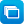# Mathematics / Year 3 / Number and Algebra / Number and place value

Curriculum content descriptions

Recall multiplication facts of two, three, five and ten and related division facts (ACMNA056)

Elaborations
• establishing multiplication facts using number sequences
General capabilities
• Numeracy Numeracy
ScOT terms

Division,  Multiplication tables

## Refine results by

Year level 3-4
Resource type
Learning area Mathematics

## Refine by topic

Related topic### Sites2See – number for primary

Selected links to a range of interactive online resources for the study of number in Foundation to Year 6 Mathematics.### Pobble arrays: find a factor

Help creatures to line up and walk through gates in equal rows and columns. Look at the given numbers of pobbles and gates, and enter the number of rows. For example, predict how many equal rows of pobbles are needed to fit 12 of them through four gates. Check your prediction. This learning object is one in a series of ...### Pobble arrays: find two factors

Help creatures to line up and walk through gates in equal rows and columns. Look at the given number of pobbles, and enter the number of gates and rows. For example, predict how many equal rows of pobbles are needed to fit 12 of them through four gates. This learning object is one in a series of three objects.### Multiplication and division

Use these resources to develop your students' understanding and use of efficient strategies for multiplication and solving problems in authentic situations. Develop strategies for a sound basis of skip counting and other examples of repeated addition. Investigate division by sharing quantities (partition) then by repeated ...### Gobbling Goblins

Welcome to 'The Toadstool Bistro', the hottest diner in Goblin Town. Maître d' Grundle is in a panic! The Gobbling Goblins, three fierce food critics, have arrived at The Toadstool Bistro and they're determined to put your kitchen to the test! Your goal is to keep the food coming for these ghastly gourmets. Use your maths ...### Mental computation: basic facts - multiplication and division

This is a teacher resource that is part of a wider series; it develops a structured strategies-based approach to mental computation of single-digit multiplication and related division facts at lower primary level. It provides 22 engaging classroom activities that will stimulate students to use appropriate mental-computation ...### reSolve: Multiplication - Domino Arrays

This sequence of two lessons explores multiplicative thinking through the use of arrays where all the parts of the array are not visible. The sequence encourages students to find the total number of items in an array by multiplication rather than counting by ones or skip counting. Connections between area, arrays and multiplication ...### Mental calculation strategies - multiplication and division

This collection of 14 digital curriculum resources focuses on assisting students to develop efficient and effective mental calculation strategies for performing multiplication and division. The collection also aims to develop and consolidate students' knowledge of multiplicative number facts. It is organised in four categories: ...### Exploring division and multiplication

This collection of 17 digital curriculum resources contains activities to consolidate and extend students' problem-solving skills in the areas of multiplication and division. The first category, arrays, allows students to visualise problems while consolidating the idea that multiplication involves repeated groups of objects. ...### What is a quarter?

What is a quarter? You get quarters when you divide a whole into four equal parts. Each one of these four parts is a quarter. Watch this great explainer produced by Monique in collaboration with ABC Splash and see how she explains quarters.### Mental computation: fractions and decimals

This is a teacher resource that is part of a wider series; it provides a structured strategies-based approach to mental computation of problems involving common and decimal fractions for primary level. It provides nine classroom activities that enable students to develop mental-computation skills in sequence by building ...### Practical Numeracy in the Kitchen and Garden

This resource provides some pick-up-and-go Numeracy activity ideas for kitchen and garden classes. It includes 4 lessons covering the three content strands, Number and algebra, Measurement and Geometry and Statistics and Probability, as well as Time.These seven learning activities, which focus on 'open-ended tasks' using a variety of tools (software) and devices (hardware), illustrate the ways in which content, pedagogy and technology can be successfully and effectively integrated in order to promote learning. In the activities, teachers use investigations in order ...### TIMES Module 3: Number and Algebra: multiplication and division - teacher guide

This is a 23-page guide for teachers. This module contains a description of suitable models for multiplication, a discussion of the type of problem phrased in words that requires multiplication for its solution, and mental and written strategies for multiplication. The use of the commutative, associative and distributive ...### Mental computation: two-digit whole numbers

This is a teacher resource that is part of a wider series; it provides a structured strategies-based approach to mental computation of two-digit whole number sums at primary level. It provides 14 classroom activities that enable students to apply appropriate mental-computation strategies to more challenging sums. The support ...### Mental computation: introduction

This is a teacher resource that is part of a wider series; it introduces a structured strategies-based approach to mental computation for primary level and provides four generic classroom activities that can be used to empower students to use, share and test strategies. The support material describes the content of the ...